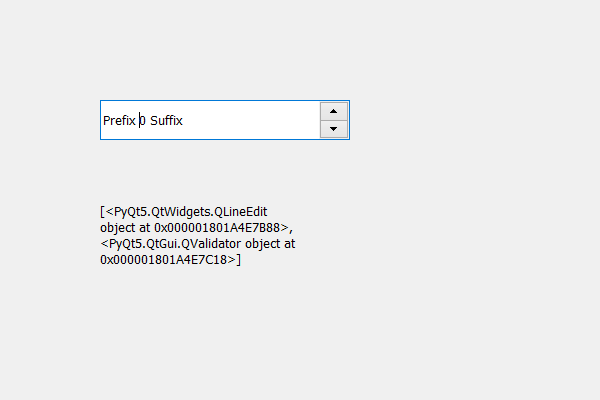Skip to content
Related Articles
PyQt5 QSpinBox – Getting list of children
• Last Updated : 17 May, 2020

In this article we will see how we can get the children of the spin box, children are basically the component of the spin box for example the spin box main component is its QLineEdit which is used to see the value and set the value.

In order to do this we use children method.

Syntax : spin_box.children()

Argument : It takes no argument

Return : It returns list

Below is the implementation

 `# importing libraries``from` `PyQt5.QtWidgets ``import` `*` `from` `PyQt5 ``import` `QtCore, QtGui``from` `PyQt5.QtGui ``import` `*` `from` `PyQt5.QtCore ``import` `*` `import` `sys`` ` ` ` `class` `Window(QMainWindow):`` ` `    ``def` `__init__(``self``):``        ``super``().__init__()`` ` `        ``# setting title``        ``self``.setWindowTitle(``"Python "``)`` ` `        ``# setting geometry``        ``self``.setGeometry(``100``, ``100``, ``600``, ``400``)`` ` `        ``# calling method``        ``self``.UiComponents()`` ` `        ``# showing all the widgets``        ``self``.show()`` ` `    ``# method for widgets``    ``def` `UiComponents(``self``):``        ``# creating spin box``        ``self``.spin ``=` `QSpinBox(``self``)`` ` `        ``# setting geometry to spin box``        ``self``.spin.setGeometry(``100``, ``100``, ``250``, ``40``)`` ` `        ``# setting prefix to spin``        ``self``.spin.setPrefix(``"Prefix "``)`` ` `        ``# setting suffix to spin``        ``self``.spin.setSuffix(``" Suffix"``)`` ` `        ``# creating a label``        ``self``.label ``=` `QLabel(``"Label "``, ``self``)`` ` `        ``# setting geometry to the label``        ``self``.label.setGeometry(``100``, ``200``, ``200``, ``70``)`` ` `        ``# getting all the children of the spin box``        ``children ``=` `self``.spin.children()`` ` `        ``# setting text to the label``        ``self``.label.setText(``str``(children))`` ` `        ``# making label multi line``        ``self``.label.setWordWrap(``True``)`` ` ` ` ` ` `# create pyqt5 app``App ``=` `QApplication(sys.argv)`` ` `# create the instance of our Window``window ``=` `Window()`` ` `# start the app``sys.exit(App.``exec``())`

Output :Attention geek! Strengthen your foundations with the Python Programming Foundation Course and learn the basics.

To begin with, your interview preparations Enhance your Data Structures concepts with the Python DS Course. And to begin with your Machine Learning Journey, join the Machine Learning – Basic Level Course

My Personal Notes arrow_drop_up